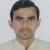true

# Dot Product of VectorsOm Prakash Mishra
24/09/2018 01

Dot or Scalar Product of Vectors

Dot Product of two vectors  A and is defines as product of magnitude of the vectors and cosine of the angle between them.

A•B = |A||B|CosT, Where T is the angle between the vectors A and B.

It is a scalar quantity

Special Cases

(I) If two vectors are perpendicular to each other their dot product is Zero.

(ii) If two vectors and B are parallel their dot product is |A||B|.

If i, j, k are unit vectorsalong X, Y, Z axis respectively then

i.i = 1

j.j = 1

k.k = 1

i.j = 0

j.k = 0

K.i = 0.

Note : Scalar product is commutative

i.e. A.B = B.A

0 Dislike
Follow 2M

Mohanrao L | 23/12/2018

the magnitude of vector to depend angle ,

## Other Lessons for You

Define static electricity. Define Eddy current. What is the difference between displacement and distance?
A

Why Electric and Magnetic Field lines can not intersects??
since tangent at any point on the field lines gives the direction of field(electric or magnetic field) so if two field lines intersects then at the intersection point we can draw two tangents from one...Enter Learning Tip Content
R

Difference between velocity and speed
SPEED VELOCITY The distance travelled the body from its initial position to final position in one second is called speed. The displacement travelled by the body in one second is called velocity It...Looking to Learn?

Find best Tutors and Coaching Centers near you on UrbanPro.

FIND NOW

Are you a Tutor or Training Institute?

Join UrbanPro Today to find students near you
X

### Find Tutors, Trainers & Institutes near you

Post requirement and connect with the tutors in your locality

• Select the best Tutor
• Book & Attend a Free Demo
• Pay and start Learning### Want to learn something New?

Find best tutors, trainers & institutes near you on UrbanPro